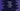# How to copy or clone a list in python### How to copy or clone a list in python :

In this tutorial, we will learn how to copy or clone a list in python. After python 3.3, one new inbuilt method was added to copy a list. We will see two different processes to copy a list in python. Method 1 can be used in both python 2 and 3. But method 2 can only be used with python 3.

### Method 1 : Using list([iterable]) :

We can pass one [iterable] to the list() constructor . If iterable is a list, it will return one list whose items are same as the input iterable. We can use this method to copy a list to one different variable. Let’s take a look :

``````first_list = []
copy_list = []

first_list.append(1)
first_list.append(2)
first_list.append(3)
first_list.append(4)
first_list.append(5)

copy_list = list(first_list)

print("Original list ",first_list)
print("Copied list ",copy_list)``````

### Output :

``````Original list  [1, 2, 3, 4, 5]
Copied list  [1, 2, 3, 4, 5]``````

### Method 2 : Using copy() method of python 3.3 :

``````first_list = []
copy_list = []

first_list.append(1)
first_list.append(2)
first_list.append(3)
first_list.append(4)
first_list.append(5)

copy_list = first_list.copy()

print("Original list ",first_list)
print("Copied list ",copy_list)``````

### Explanation :

From python 3.3 , one new simple method was added to copy a list to a different list. In the above program, we are using this method to copy one list. Remember to check your python version before running this program. The output will be :

``````Original list  [1, 2, 3, 4, 5]
Copied list  [1, 2, 3, 4, 5]``````

So, the full list is copied to the variable copylist_.